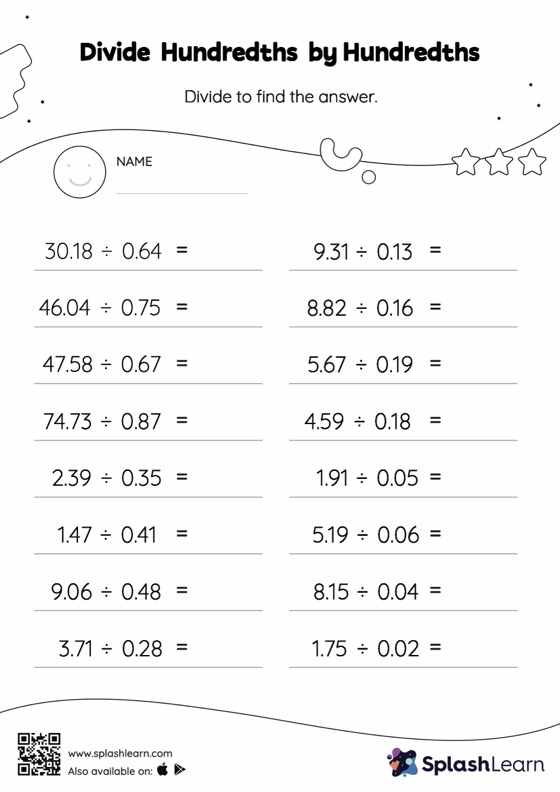# Divide Hundredths by Hundredths with Remainder: Horizontal Division Worksheet

Home > Divide Hundredths by Hundredths with Remainder: Horizontal DivisionCan your young mathematician divide hundredths by hundredths with remainder? That's exactly what they do here. When students divide two decimals, they first divide as if there is no decimal. Depending on the number of decimal digits in each number, they add a decimal point at the appropriate location in the quotient. Students practice this concept in great detail with this divide hundredths by hundredths with remainder worksheet.